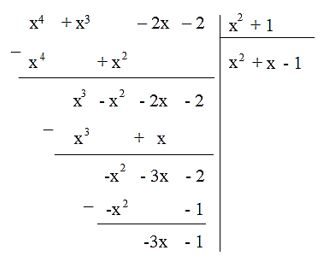## Detailed instructions for solving Problem 7.44

Solution method

* How to add (subtract) 2 polynomials:

Method 1: Remove the brackets and group the terms of the same degree.

Method 2: Set the addition (subtract) so that the terms of the same degree are placed in the same column and then add (subtract) by each column.

* How to multiply 2 polynomials:

Method 1: To multiply a polynomial by a polynomial, we multiply each term of this polynomial by each term of the other polynomial and then add the products together.

Method 2: Set the multiplier:

+ Multiply each term in the lower row by the polynomial in the upper line and write the result in a separate line.

+ Write the lines so that the terms of the same order are in the same column to perform the addition by column.

* To divide polynomial A by polynomial B, we do the following:

Step 1: Set the divisibility similar to dividing two natural numbers. Divide the highest-order term of A by the highest-order term of B.

Step 2: Take A minus the product of B with the new quotient obtained in step 1

Step 3: Divide the highest order term of the first remainder by the highest term of B

Step 4: Subtract the product B from the first remainder with the quotient obtained in step 3

Step 5: Do the same as above

When the last remainder is of degree less than the degree of B, the division ends.

Detailed explanation

a) We have:

B = (A + B) – A

= (x3 + 3x + 1) – (x4 + x3 – 2x – 2)

= x3 + 3x + 1 – x4 – x3 + 2x + 2

= – x4 + (x3 – x3) + (3x + 2x) + (1 + 2)

= – x4 + 5x + 3

b) C = (A – C) – A

= x5 – (x4 + x3 – 2x – 2)

= x5 – x4 – x3 + 2x + 2)

c) D = (2x3 – 3) . A

= (2x3 – 3) . (x4 + x3 – 2x – 2)

= 2x3 . (x4 + x3 – 2x – 2) + (-3) .(x .)4 + x3 – 2x – 2)

= 2x3 . x4 + 2x3 . x3 + 2x3 . (-2x) + 2x3 . (-2) + (-3). x4 + (-3) . x3 + (-3). (-2x) + (-3). (-2)

= 2x7 + 2x6 – 4x4 – 4x3 – 3x4 – 3x3 + 6x + 6

= 2x7 + 2x6 + (-4x4 – 3x4) + (-4x3 – 3x3) + 6x + 6

= 2x7 + 2x6 – 7x4 – 7x3 + 6x + 6

d) P = A : (x+1) = (x4 + x3 – 2x – 2) : (x + 1)So P = x3 – 2

e) Q = A : (x2 + 1)

If A divided by the polynomial x2 + 1 has no remainder, then there is a satisfying polynomial Q

We perform division (x4 + x3 – 2x – 2) : (x2 + 1)Since division has remainders, there is no satisfying polynomial Q

–>

— *****

### Solution 7.45 page 46 Math textbook 7 Connecting knowledge volume 2 – KNTT

Let the polynomial P(x). Explain why if there is a polynomial Q(x) such that P(x) = (x – 3). Q(x) (i.e. P(x) is divisible by x – 3) then x = 3 is a solution of P(x)

## Detailed instructions for solving Problem 7.45

Solution method

The root of the polynomial variable x is the value of x where the polynomial has a value of 0.

Detailed explanation

Because at x = 3 then P(x) = (3 – 3) . Q(x) = 0. Q(x) = 0 so x = 3 is a solution of the polynomial P(x)

–>

— *****

### Solution 7.46 page 46 Math textbook 7 Connecting knowledge volume 2 – KNTT

Two friends Round and Square argue with each other as follows:## Detailed instructions for solving problems 7.46

Solution method

The sum of polynomials is a polynomial of degree no greater than the degree of the component polynomials

Detailed explanation

Round is true, Square is false because the sum of the polynomials is a polynomial of degree not greater than the degree of the component polynomials.

Polynomial M(x) = x3 + 1 can be written as the sum of two quadratic polynomials whose highest coefficients are two opposite numbers.

For example:

x3 + 1 = (x4 + 1) + (-x4 + x3)

–>

— *****